# Pytorch入门教程05-梯度下降算法2020年10月4日07:03:08

2 2875字阅读9分35秒

## 简介

• 梯度下降法的简单介绍;
• 手动实现梯度下降法;
• 使用Pytroch自动实现梯度下降, 结合backward实现.

## 梯度下降介绍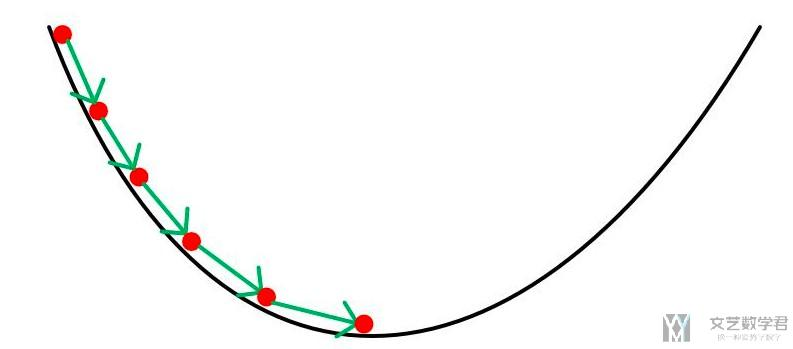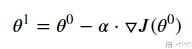## 人工实现梯度下降法

### 生成测试数据

1. # 定义数据集合
2. X = np.arange(0,10,0.1, dtype=np.float32)
3. Y = 2*X + 2*np.random.random(100)

1. # 可视化数据集
2. fig = plt.figure(figsize=(12,8))
4. ax.scatter(X,Y)
5. fig.show()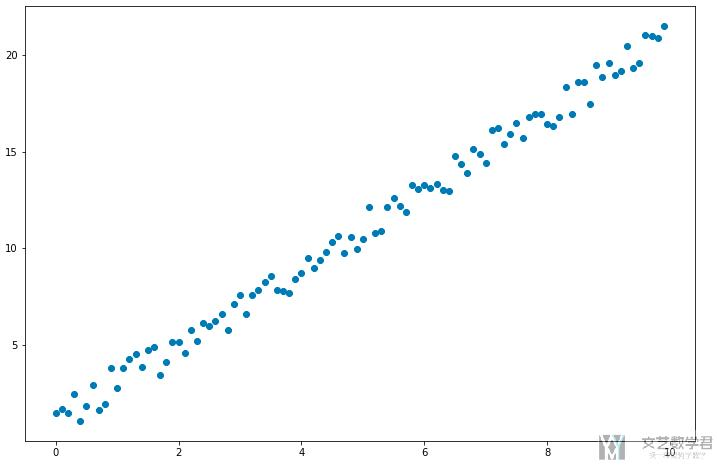### 定义损失函数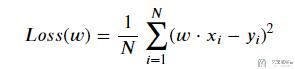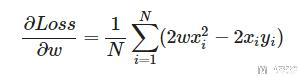1. #返回dJ/dw
3.     """计算梯度
4.     """
5.     return np.mean(2*w*x*x-2*x*y)

### 梯度下降

1. 随机初始化一个w的值;
2. 在该w 下进行正向传播, 得到所有x的预测值 y_pre;
3. 通过实际的值y和预测值y_pre计算损失;
4. 通过损失计算梯度dw;
5. 更新w = w-lr*dw, 其中lr为步长(learning rate), 可自定义具体的值;
6. 重复步骤2-5, 直到损失降到较小位置;

1. # 我们先定义一些变量
2. def forward(x):
3.     return w * x
4. def loss(y, y_pred):
5.     return ((y_pred - y)**2).mean()
6. w = 0.0 # 初始化系数
7. # 定义步长和迭代次数
8. learning_rate = 0.001
9. n_iters = 100

1. for epoch in range(n_iters):
2.     # 彰显传播
3.     y_pred = forward(X)
4.     #计算损失
5.     # l = loss(Y, y_pred)
6.     #计算梯度
7.     dw = gradient(X, Y, w)
8.     #更新权重 w
9.     w = w - learning_rate * dw
10.     if epoch % 20 == 0:
11.         print(f'epoch {epoch+1}: w = {w:.3f}, loss = {l:.8f}')
12. print(f'根据训练模型预测，当 x=7 时，y 的值为： {forward(7):.3f}')
13. """
14. epoch 1: w = 0.142, loss = 0.06237932
15. epoch 21: w = 1.639, loss = 0.06237932
16. epoch 41: w = 2.024, loss = 0.06237932
17. epoch 61: w = 2.123, loss = 0.06237932
18. epoch 81: w = 2.148, loss = 0.06237932
19. 根据训练模型预测，当 x=7 时，y 的值为： 15.084
20. """

### 结果可视化

1. # 绘制预测曲线
2. y_pre = forward(X)
3. fig = plt.figure(figsize=(12,8))
5. ax.scatter(X,Y)
6. ax.plot(X, y_pre, 'g-',  lw=3)
7. fig.show()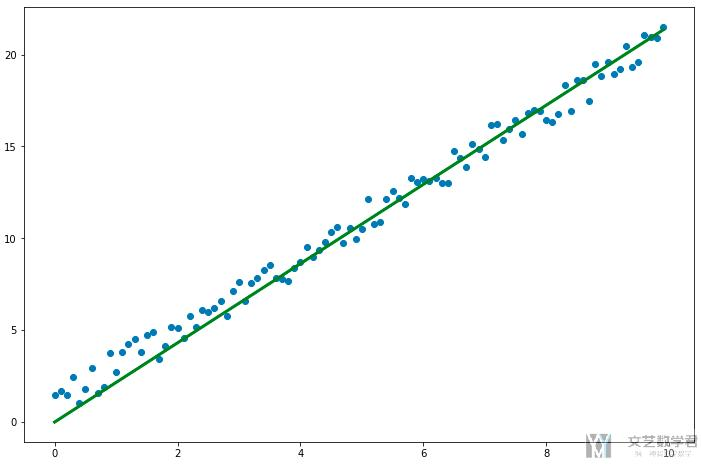## Pytorch中的梯度下降法

1. X = np.arange(0,10,0.1, dtype=np.float32)
2. Y = 2*X + 2*np.random.random(100)
3. X_tensor = torch.from_numpy(X)
4. Y_tensor = torch.from_numpy(Y)
5. w = torch.tensor(0.0, dtype=torch.float32, requires_grad=True)
6. learning_rate = 0.001
7. n_iters = 100

1. for epoch in range(n_iters):
2.     y_pred = forward(X_tensor)
3.     l = loss(Y_tensor, y_pred) # 求误差
4.     l.backward() # 求梯度
6.         w.data = w.data - learning_rate * w.grad
7.     # 清空梯度
9.     if epoch % 20 == 0:
10.         print(f'epoch {epoch+1}: w = {w.item():.3f}, loss = {l.item():.3f}')
11. print(f'根据训练模型预测, 当x=5时, y的值为： {forward(5):.3f}')
12. """
13. epoch 1: w = 0.140, loss = 149.477
14. epoch 21: w = 1.618, loss = 10.344
15. epoch 41: w = 1.999, loss = 1.151
16. epoch 61: w = 2.096, loss = 0.544
17. epoch 81: w = 2.121, loss = 0.504
18. 根据训练模型预测, 当x=5时, y的值为： 10.638
19. """

• 微信公众号
• 关注微信公众号
•• QQ群
• 我们的QQ群号
•• 本文由 发表于 2020年10月4日07:03:08
• 转载请务必保留本文链接：https://mathpretty.com/12511.html